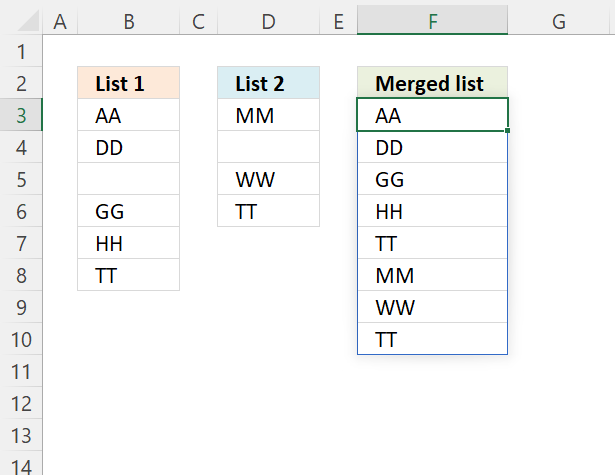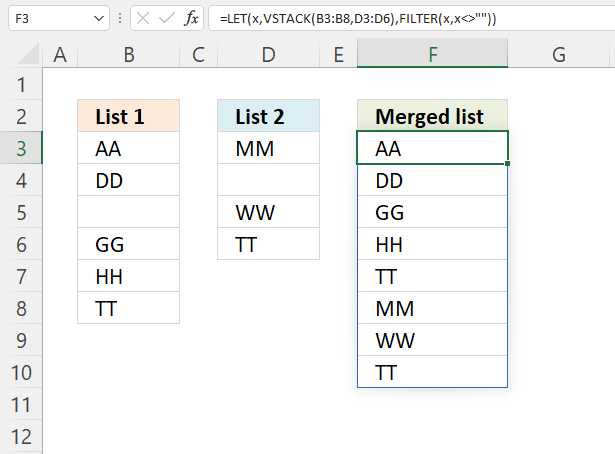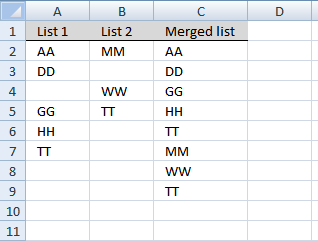Author: Oscar Cronquist Article last updated on July 01, 2022This article demonstrates two formulas, they both accomplish the same thing. The Excel 365 formula is much smaller and is easy to modify if more columns are needed in the formula.

## Merge two columns with possible blank cells - Excel 365This example works only in Excel 365, it contains three functions only available for Excel 365: LET, VSTACK and FILTER. A dynamic array formula is entered as a regular formula, however, it spills values to adjacent cells automatically as far as needed.

Dynamic array formula in cell F3:

=LET(x, VSTACK(B3:B8, D3:D6), FILTER(x,x<>""))

### Explaining formula

#### Step 1 - Stack cell ranges vertically

The VSTACK function combines cell ranges or arrays. Joins data to the first blank cell at the bottom of a cell range or array (vertical stacking).

VSTACK(array1, [array2], ...)

VSTACK(B3:B8,D3:D6)

becomes

VSTACK({"AA"; "DD"; 0; "GG"; "HH"; "TT"}, {"MM"; 0; "WW"; "TT"})

and returns

{"AA"; "DD"; 0; "GG"; "HH"; "TT"; "MM"; 0; "WW"; "TT"}.

#### Step 2 - Remove blanks

The FILTER function filter values/rows based on a condition or criteria.

FILTER(arrayinclude, [if_empty])

FILTER(VSTACK(B3:B8,D3:D6),VSTACK(B3:B8,D3:D6)<>"")

becomes

FILTER(VSTACK(B3:B8,D3:D6),{"AA"; "DD"; 0; "GG"; "HH"; "TT"; "MM"; 0; "WW"; "TT"}<>"")

becomes

FILTER(VSTACK(B3:B8,D3:D6), {TRUE; TRUE; FALSE; TRUE; TRUE; TRUE; TRUE; FALSE; TRUE; TRUE})

becomes

FILTER({"AA"; "DD"; 0; "GG"; "HH"; "TT"; "MM"; 0; "WW"; "TT"}, {TRUE; TRUE; FALSE; TRUE; TRUE; TRUE; TRUE; FALSE; TRUE; TRUE})

and returns

{"AA"; "DD"; "GG"; "HH"; "TT"; "MM"; "WW"; "TT"}.

#### Step 3 - Shorten formula

The LET function lets you name intermediate calculation results which can shorten formulas considerably and improve performance.

LET(name1name_value1calculation_or_name2, [name_value2calculation_or_name3...])

FILTER(VSTACK(B3:B8,D3:D6),VSTACK(B3:B8,D3:D6)<>"")

VSTACK(B3:B8,D3:D6) is repeated twice in the formula above. I will name this intermediate calculation x.

LET(x,VSTACK(B3:B8,D3:D6),FILTER(x,x<>""))

The result is a smaller formula.

## Merge two columns with possible blank cells - earlier versions

### Question:

I do have one question:

Let's say my "List 1" is auto updated and the number of entries in this list will fluctuate. Since the number of entries fluctuates, I would like to select a larger range than I actually have data in currently. The issue is when I make my "List 1" larger than the number of entries, the rows that don't currently have data in them, show up on my combined list as zeros.

So my question is, is there a way to adjust the formula so that when it looks at "List 1" for example, it skips over blank cells and continues to combine the list with "List 2".

(You can find the question here: Merge two columns into one list in excel)

The array formula below removes blank cells. Another method is to use dynamic named ranges.Array formula in C2:

=IFERROR(INDEX(List1, SMALL(IF(ISBLANK(List1), "", ROW(List1)-MIN(ROW(List1))+1), ROW(A1))), IFERROR(INDEX(List2, SMALL(IF(ISBLANK(List2), "", ROW(List2)-MIN(ROW(List2))+1), ROW(A1)-SUMPRODUCT(--NOT((ISBLANK(List1)))))), ""))

Recommended post:

Recommended articles

Combine cell ranges ignore blank cells
The image above demonstrates a user defined function that merges up to 255 cell ranges and removes blanks. I will also […]

This is an array formula, here is how to enter it. Type the formula in cell C2, press and hold CTRL + SHIFT simultaneously. Press Enter once. Release all keys. If you did it correctly, you now have curly brackets before and after the formula.

Copy cell C2 and paste it to cells below, as far as needed.

This example merges two columns into one column using an array formula. If you are looking for merging two data lists with criteria, check this post: Merge lists with criteria

### Named ranges

List1 (A2:A7)
List2 (B2:B5)

Recommended article:

Recommended articles

Merge three columns into one list
The above image demonstrates a formula that adds values in three different columns into one column. Formula in H2: =IFERROR(INDEX(\$B\$3:\$B\$7, […]

### Explaining array formula in cell C8

#### Step 1 - Understand relative and absolute cell references

In cell C2 the formula is:

=IFERROR(INDEX(List1, SMALL(IF(ISBLANK(List1), "", ROW(List1)-MIN(ROW(List1))+1), ROW(A1))), IFERROR(INDEX(List2, SMALL(IF(ISBLANK(List2), "", ROW(List2)-MIN(ROW(List2))+1), ROW(A1)-SUMPRODUCT(--NOT((ISBLANK(List1)))))), ""))

In cell C8 the formula is:

=IFERROR(INDEX(List1, SMALL(IF(ISBLANK(List1), "", ROW(List1)-MIN(ROW(List1))+1), ROW(A7))), IFERROR(INDEX(List2, SMALL(IF(ISBLANK(List2), "", ROW(List2)-MIN(ROW(List2))+1), ROW(A7)-SUMPRODUCT(--NOT((ISBLANK(List1)))))), ""))

Recommended articles

How to use absolute and relative references
What is a reference in Excel? Excel has an A1 reference style meaning columns are named letters A to XFD […]

#### Step 1 - Find cells containing a value in List 1 and return row numbers in an array

=IFERROR(INDEX(List1, SMALL(IF(ISBLANK(List1), "", ROW(List1)-MIN(ROW(List1))+1), ROW(A7))), IFERROR(INDEX(List2, SMALL(IF(ISBLANK(List2), "", ROW(List2)-MIN(ROW(List2))+1), ROW(A7)-SUMPRODUCT(--NOT((ISBLANK(List1)))))), ""))

IF(ISBLANK(List1), "", ROW(List1)-MIN(ROW(List1))+1)

becomes

IF(ISBLANK({"AA";"DD";"";"GG";"HH";"TT"}), "", ROW(A2:A7)-MIN(ROW(A2:A7))+1)

becomes

IF({FALSE;FALSE;TRUE;FALSE;FALSE;FALSE}, "", {2;3;4;5;6;7}-MIN({2;3;4;5;6;7})+1)

becomes

IF({FALSE;FALSE;TRUE;FALSE;FALSE;FALSE}, "", {2;3;4;5;6;7}-2+1)

becomes

IF({FALSE;FALSE;TRUE;FALSE;FALSE;FALSE}, "", {1;2;3;4;5;6})

and returns

{1;2;"";4;5;6}

Recommended articles

How to use the IF function
Checks if a logical expression is met. Returns a specific value if TRUE and another specific value if FALSE.

#### Step 2 - Return the k-th smallest row number

=IFERROR(INDEX(List1, SMALL(IF(ISBLANK(List1), "", ROW(List1)-MIN(ROW(List1))+1), ROW(A7))), IFERROR(INDEX(List2, SMALL(IF(ISBLANK(List2), "", ROW(List2)-MIN(ROW(List2))+1), ROW(A7)-SUMPRODUCT(--NOT((ISBLANK(List1)))))), ""))

SMALL(array,k) Returns the k-th smallest number in this data set.

SMALL(IF(ISBLANK(List1), "", ROW(List1)-MIN(ROW(List1))+1), ROW(A7))

becomes

SMALL({1;2;"";4;5;6}, 8 )

and returns #NUM

Recommended articles

How to use the SMALL function
The SMALL function lets you extract a number in a cell range based on how small it is compared to the other numbers in the group.

#### Step 3 - Return a value of the cell at the intersection of a particular row and column

=IFERROR(INDEX(List1, SMALL(IF(ISBLANK(List1), "", ROW(List1)-MIN(ROW(List1))+1), ROW(A7))), IFERROR(INDEX(List2, SMALL(IF(ISBLANK(List2), "", ROW(List2)-MIN(ROW(List2))+1), ROW(A7)-SUMPRODUCT(--NOT((ISBLANK(List1)))))), ""))

INDEX(array,row_num,[column_num]) returns a value or reference of the cell at the intersection of a particular row and column, in a given range

INDEX(List1, SMALL(IF(ISBLANK(List1), "", ROW(List1)-MIN(ROW(List1))+1), ROW(A7)))

becomes

INDEX(List1, #NUM)

and returns

#NUM

Recommended articles

How to use the INDEX function
Gets a value in a specific cell range based on a row and column number.

#### Step 4 - Check if formula returns an error

=IFERROR(INDEX(List1, SMALL(IF(ISBLANK(List1), "", ROW(List1)-MIN(ROW(List1))+1), ROW(A7))), IFERROR(INDEX(List2, SMALL(IF(ISBLANK(List2), "", ROW(List2)-MIN(ROW(List2))+1), ROW(A7)-SUMPRODUCT(--NOT((ISBLANK(List1)))))), ""))

becomes

=IFERROR(#NUM, IFERROR(INDEX(List2, SMALL(IF(ISBLANK(List2), "", ROW(List2)-MIN(ROW(List2))+1), ROW(A7)-SUMPRODUCT(--NOT((ISBLANK(List1)))))), ""))

becomes

IFERROR(INDEX(List2, SMALL(IF(ISBLANK(List2), "", ROW(List2)-MIN(ROW(List2))+1), ROW(A7)-SUMPRODUCT(--NOT((ISBLANK(List1)))))), "")

Recommended articles

How to use the IFERROR function
The IFERROR function lets you catch most errors in Excel formulas. It was introduced in Excel 2007. In previous Excel […]

#### Step 5 - Find cells containing a value in List 2

IFERROR(INDEX(List2, SMALL(IF(ISBLANK(List2), "", ROW(List2)-MIN(ROW(List2))+1), ROW(A7)-SUMPRODUCT(--NOT((ISBLANK(List1)))))), "")

IF(ISBLANK(List2), "", ROW(List2)-MIN(ROW(List2))+1)

becomes

IF(ISBLANK({"MM";"";"WW";"TT"}), "", {2;3;4;5}-MIN({2;3;4;5})+1)

becomes

IF({FALSE;TRUE;FALSE;FALSE}), "", {1;2;3;4})

and returns

{1;"";3;4}

#### Step 6 - Return the k-th smallest row number

IFERROR(INDEX(List2, SMALL(IF(ISBLANK(List2), "", ROW(List2)-MIN(ROW(List2))+1), ROW(A8)-SUMPRODUCT(--NOT((ISBLANK(List1)))))), "")

SMALL(array,k) Returns the k-th smallest number in this data set.

SMALL(IF(ISBLANK(List2), "", ROW(List2)-MIN(ROW(List2))+1), ROW(A8)-SUMPRODUCT(--NOT((ISBLANK(List1)))))

becomes

SMALL({1;"";3;4}, ROW(A7)-SUMPRODUCT(--NOT((ISBLANK(List1)))))

becomes

SMALL({1;"";3;4}, 7-SUMPRODUCT(--NOT((ISBLANK({"AA";"DD";"";"GG";"HH";"TT"})))))

becomes

SMALL({1;"";3;4}, 7-SUMPRODUCT(--NOT({FALSE;FALSE;TRUE;FALSE;FALSE;FALSE})))

becomes

SMALL({1;"";3;4}, 7-SUMPRODUCT(--{TRUE;TRUE;FALSE;TRUE;TRUE;TRUE}))

becomes

SMALL({1;"";3;4}, 7-SUMPRODUCT({1;1;0;1;1;1})

becomes

SMALL({1;"";3;4}, 7-5)

becomes

SMALL({1;"";3;4}, 2)

and returns 3.

#### Step 6 - Return a value of the cell at the intersection of a particular row and column

IFERROR(INDEX(List2, SMALL(IF(ISBLANK(List2), "", ROW(List2)-MIN(ROW(List2))+1), ROW(A8)-SUMPRODUCT(--NOT((ISBLANK(List1)))))), "")

becomes

IFERROR(INDEX(List2, 3), "")

becomes

IFERROR(INDEX({"MM";0;"WW";"TT"}, 3), "")

becomes

IFERROR("WW", "")

and returns WW.